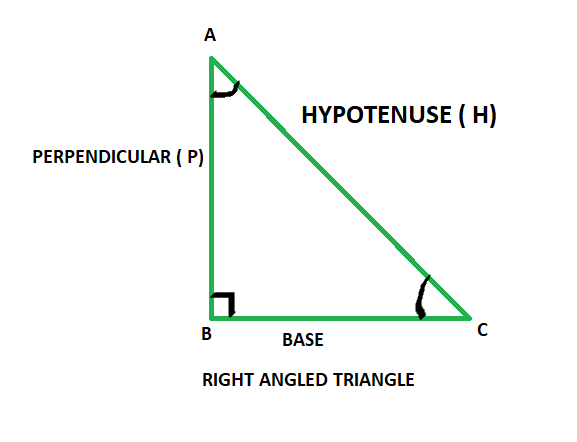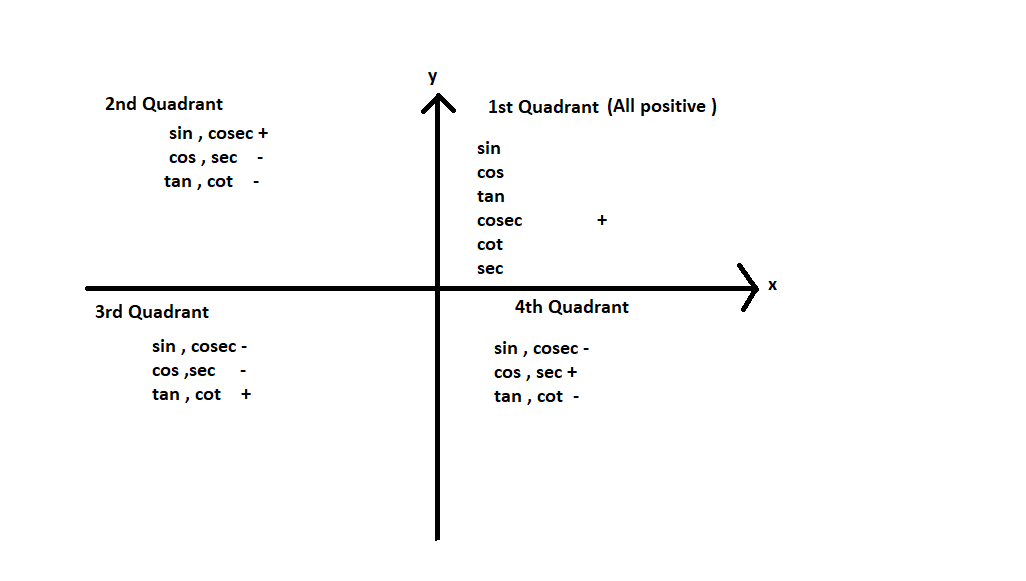# Find the value of sin 120° cosec 240° tan 210°

• Last Updated : 15 Nov, 2021

Trigonometry is a discipline of mathematics that studies the relationships between the lengths of the sides and angles of a right-angled triangle. Trigonometric functions, also known as goniometric functions, angle functions, or circular functions, are functions that establish the relationship between an angle to the ratio of two of the sides of a right-angled triangle. The six main trigonometric functions are sine, cosine, tangent, cotangent, secant, or cosecant.

Angles defined by the ratios of trigonometric functions are known as trigonometry angles. Trigonometric angles represent trigonometric functions. The value of the angle can be anywhere between 0-360°.

Hey! Looking for some great resources suitable for young ones? You've come to the right place. Check out our self-paced courses designed for students of grades I-XII

Start with topics like Python, HTML, ML, and learn to make some games and apps all with the help of our expertly designed content! So students worry no more, because GeeksforGeeks School is now here!RIGHT ANGLE TRIANGLE

As given in the above figure in a right-angled triangle:

• Hypotenuse: The side opposite to the right angle is the hypotenuse, It is the longest side in a right-angled triangle and opposite to the 90° angle.
• Base: The side on which angle C lies is known as the base.
• Perpendicular: It is the side opposite to angle C in consideration.

### Trigonometric Functions

Trigonometry has 6 basic trigonometric functions, they are sine, cosine, tangent, cosecant, secant, and cotangent. Now let’s look into the trigonometric functions. The six trigonometric functions are as follows:

• sine: It is defined as the ratio of perpendicular and hypotenuse and It is represented as sin θ
• cosine: It is defined as the ratio of base and hypotenuse and it is represented as cos θ
• tangent: It is defined as the ratio of sine and cosine of an angle. Thus the definition of tangent comes out to be the ratio of perpendicular and base and is represented as tan θ
• cosecant: It is the reciprocal of sin θ and is represented as cosec θ.
• secant: It is the reciprocal of cos θ and is represented as sec θ.
• cotangent: It is the reciprocal of tan θ and is represented as cot θ.

According to above given image, Trigonometric Ratios are

• Sin θ = Perpendicular / Hypotenuse = AB/AC
• Cosine θ = Base / Hypotenuse = BC / AC
• Tangent θ = Perpendicular / Base = AB / BC
• Cosecant θ = Hypotenuse / Perpendicular = AC/AB
• Secant θ = Hypotenuse / Base = AC/BC
• Cotangent θ = Base / Perpendicular = BC/AB

Reciprocal Identities

Sin θ = 1/ Cosec θ                    Or        Cosec θ = 1/ Sin θ

Cos θ = 1/ Sec θ                       Or         Sec θ = 1 / Cos θ

Cot θ = 1 / Tan θ                     Or         Tan θ = 1 / Cot θ

Cot θ = Cos θ / Sin θ               Or          Tan θ = Sin θ / Cos θ

Tan θ.Cot θ = 1

Values of Trigonometric Ratios

Trigonometric Identities of Complementary and Supplementary Angles

• Complementary Angles: Pair of angles whose sum is equal to 90°
• Supplementary Angles: Pair of angles whose sum is equal to 180°

Identities of Complementary angles are

sin (90° – θ) = cos θ

cos (90° – θ) = sin θ

tan (90° – θ) = cot θ

cot (90° – θ) = tan θ

sec (90° – θ) = cosec θ

cosec (90° – θ) = sec θ

Identities of supplementary angles

sin (180° – θ) = sin θ

cos (180° – θ) = – cos θ

tan (180° – θ) = – tan θ

cot  (180° – θ) = – cot θ

sec (180° – θ) = – sec θ

cosec (180° – θ) = – cosec θ### Find the value of sin 120° cosec 240° tan 210°

Solution:

Here we have sin 120° cosec 240° tan 210°

We can write as Sin (90+30) cosec (180+60) tan (180+30)  { as per the quadrants and trigonometric values }

=  Sin 30° × Cosec 60° × ( -Tan 30°)

=  1/2 × 2/√3 × -1√3

= – 1/3

The value of sin 120° cosec 240° tan 210° is – 1/3

### Similar Questions

Question 1: What is the exact value of sin 270?

Solution:

Here sin is positive only in 1st and 2nd Quadrant.

270° does not lies in 1st and 2nd Quadrant.

Therefore     sin (360° – θ) = – sin θ

sin (270°) = sin (360° – 90°)

sin (270°) = – sin (90°)

sin (270°) = – 1

So the exact value of sin 270 is -1

Question 2: Evaluate (Sin 30° – Sin 90° + 2 Cos 0°) / Tan 30° Tan 60°?

Solution:

Here we have (Sin 45° – Sin 90° + 2 Cos 0°) /  Tan 45° Tan 60°

As per the trigonometric values

(Sin 45° – Sin 90° +2 Cos 0°) /  Tan 45° Tan 60°

= (1/√2 – 1 + 2 × 1) /  1 × √3

= (1/√2 – 1 + 2) / √3

= (1/√2 + 1) / √3

= (1+√2 / √2) / √3

My Personal Notes arrow_drop_up